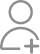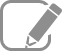Take Class 12 Tuition from the Best Tutors

•Affordable fees
•1-1 or Group class
•Flexible Timings
•Verified Tutors

Search in

# Following are the balances of current assets and current liabilities of X ltd., Cash Rs.20,000; Outstanding Expenses Rs.5000; Creditors Rs.15,000; Debtors Rs.50,000; Short Term Loans Rs.4000; Short Term Investments Rs.30,000; Long term loans Rs.1,00,000; Stock Rs.70,000. Calculate the gross and net working capital of X Ltd.Follow 1AnswerGross working capital is sum of all current assets of the firm so we need to add all current assets to calculate gross working capital as follows. Gross working capital = Cash + Debtors + Short Term Inv + Stocks = Rs (20000+50000+30000+70000) = Rs 170000. Net working capital = Current Assets - Current...

Gross working capital is sum of all current assets of the firm so we need to add all current assets to calculate gross working capital as follows.

Gross working capital

= Cash + Debtors + Short Term Inv + Stocks

= Rs (20000+50000+30000+70000)

= Rs 170000.

Net working capital

= Current Assets - Current Liabilities

= Rs 170000 - (5000 + 15000 + 4000)

= Rs 146000.

Related Questions

Explain in detail objectives and three important Primary functions of NABARD.
1. Integrated Rural Development Programme IRDP is a scheme devised by Government of India for generating self-employment opportunities in the rural sector and for the economic development of rural areas....Now ask question in any of the 1000+ Categories, and get Answers from Tutors and Trainers on UrbanPro.com

Find Class 12 Tuition near you

Looking for Class 12 Tuition ?

Learn from the Best Tutors on UrbanPro

Are you a Tutor or Training Institute?

Join UrbanPro Today to find students near you
X

### Looking for Class 12 Tuition Classes?

The best tutors for Class 12 Tuition Classes are on UrbanPro

• Select the best Tutor
• Book & Attend a Free Demo
• Pay and start Learning### Take Class 12 Tuition with the Best Tutors

The best Tutors for Class 12 Tuition Classes are on UrbanPro